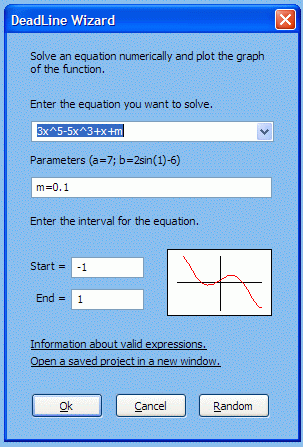Enter the equation, the interval where you want to solve it and the parameters if you want solve parametric equations. Starting with DeadLine 2.30, you can preview the graph in DeadLine Wizard, so you can tweak the interval and the parameters faster.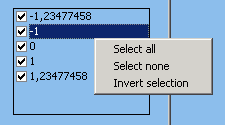DeadLine automatically displays the real roots of the equation in a scrollable list. To locate a root on the graph, select it from the list.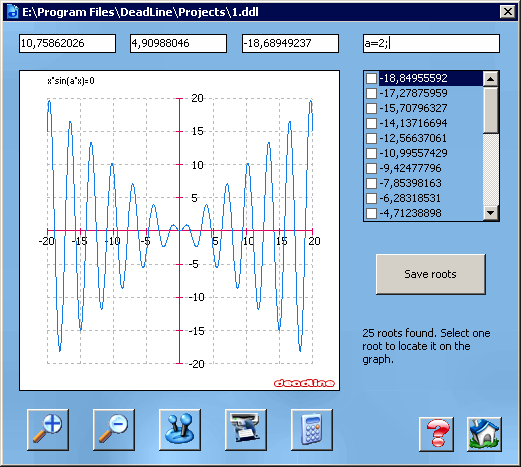Graphs can help you locate the intervals that contain roots. Once you know where to search for the roots, DeadLine finds them for you. You can also solve parametric equations.
Plot graphs in full-screen view, zoom in and zoom out, change the interval, customize the colors and save the graphs.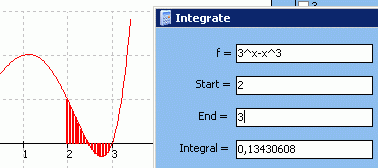Integrate a function numerically. The graph is shaded to better visualize the selected area.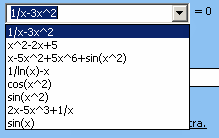DeadLine saves the most recently used equations. To reopen an equation, select the name from the list or type the first characters.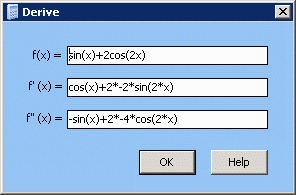Find the first two derivatives of a function.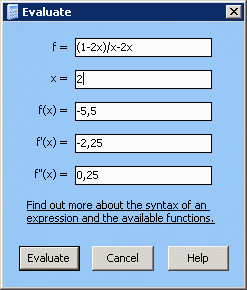Evaluate a function and the first two derivatives at a certain value.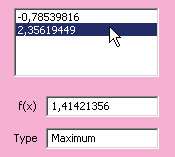Optimize functions: find local minima and local maxima, locate on the graph, and save extrema.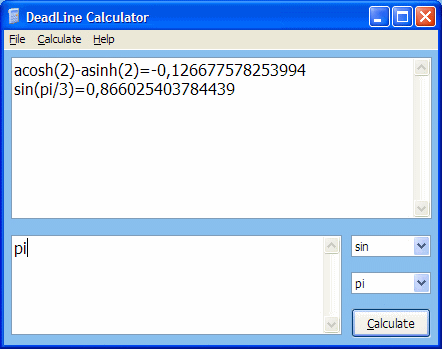Perform simple calculations, define your own functions and constants with DeadLine Calculator. It's faster than the standard calculator in Windows.
DeadLine OnLine - free equation solver. Copyright 2003-2007 Ionut Alex. Chitu. | Contact | Sitemap